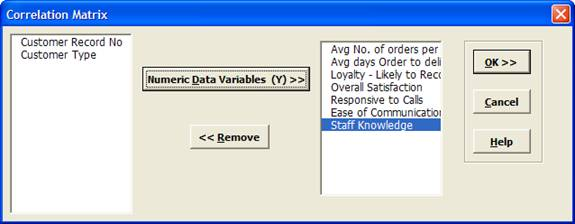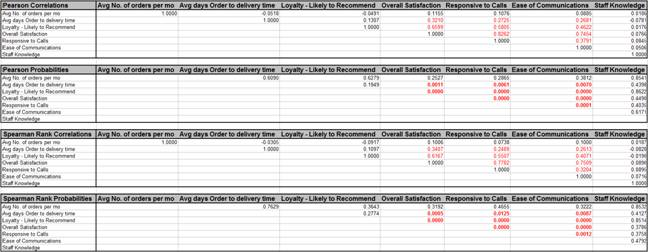Include Top

# How Do I create a Correlation Matrix in Excel Using SigmaXL?

The correlation matrix complements the scatterplot matrix by quantifying the degree of association. The following table shows the approximate relationship between r, R-squared, and degree of association:

 Pearson Correlation Coefficient (r) R-Squared (%) Degree of association 0.9 <= |r| <= 1 > 80% Strong 0.7 <= |r| <= 0.9 50% to 80% Moderate |r| < 0.7 < 50% Weak Pearson Probability, p > 0.05 None

1. Open Customer Data.xlsx. Click Sheet 1 tab (or press F4 to activate last worksheet). Click SigmaXL > Statistical Tools > Correlation Matrix. Ensure that entire data table is selected. If not, check Use Entire Data Table. Click Next.
2. Select the variable Avg No. of orders per month; shift-click on Staff Knowledge and click Numeric Data Variables (Y) >> as shown:1. Click OK. Resulting Correlation Matrix is shown:

2.Correlations highlighted in red are considered significant (p-values < .05). The corresponding correlation coefficients above the p-values are also highlighted in red. (Compare these to the Scatterplot Matrix.)

Note that Spearman’s Rank Correlation complements Pearson’s Correlation, in that it provides a robust measure of association. Spearman’s rank is based on correlated ranks, which are not sensitive to outliers.

# Web Demos

Our CTO and Co-Founder, John Noguera, regularly hosts free Web Demos featuring SigmaXL and DiscoverSim